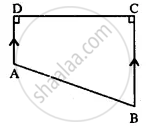# The Following Figure Shows the Cross-section Abcd of a Swimming Pool Which is a Trapezium in Shape. If the Width Dc, of the Swimming Pool, is 6.4cm, Depth (Ad) at the Shallow - Mathematics

Sum

The following figure shows the cross-section ABCD of a swimming pool which is a trapezium in shape.
If the width DC, of the swimming pool, is 6.4cm, depth (AD) at the shallow end is 80 cm and depth (BC) at the deepest end is 2.4m, find Its area of the cross-section.#### Solution

Area of the cross-section = Area of trapezium ABCD

= 1/2 ("Sum of parallel sides") xx "height"

= 1/2 (80 + 240) xx 6.4

= (320) (3.2) = (32) (32)

= 1024 cm2 or = 10.24 sq.m.

Concept: Area of Trapezium
Is there an error in this question or solution?

#### APPEARS IN

Selina Concise Mathematics Class 8 ICSE
Chapter 20 Area of a Trapezium and a Polygon
Exercise 20 (C) | Q 1 | Page 232